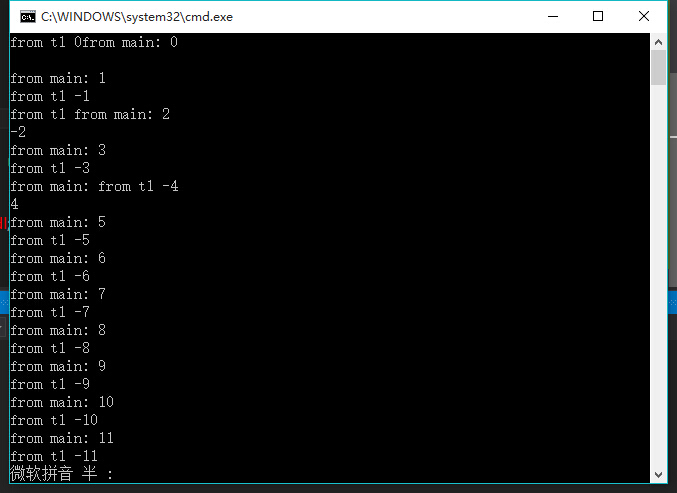C++ 线程和仿函数的问题？？
`````` #include<iostream>
using namespace std;
void function_1()
{
std::cout << "Hello,world!" << std::endl;
}
class Fctor {                                      //仿函数
public:
void operator()() {                    //对（）进行重载
for (int i = 0; i > -100; i--) {
cout << "from t1 " << i << endl;
}
}
};
int main() {
//Fctor fct;
std::thread t1((Fctor()));         //t1  starts  running. 实例化一个线程对象t1
try {
for (int i = 0; i < 100; i++)
cout << "from main: " << i << endl;
}
catch (...) {
t1.join();
throw;
}
t1.join();
//t1.detach();
return 0;
}
``````3个回答4 年多之前 回复

Fctor() ：可以理解成函数名字为Fctor的函数。
(Fctor()): 作为表达式。